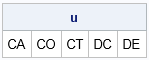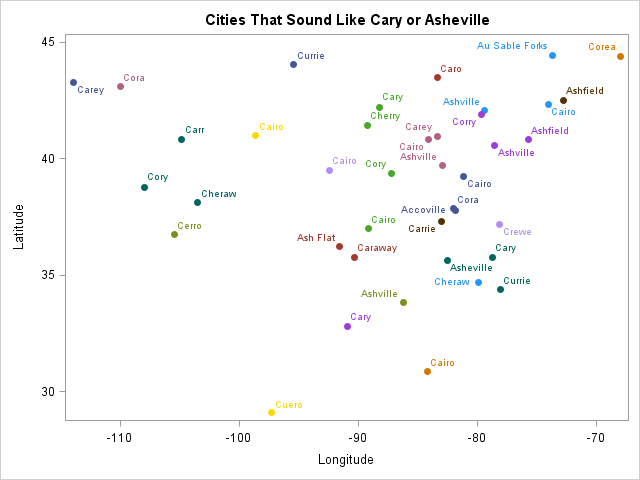The WHERE clause in SAS is a powerful mechanism for selecting observations as you read or write a data set. The WHERE clause supports many operators, including the IN operator, which enables you to compactly specify multiple conditions for a categorical variable.

A common use of the IN operator is to specify a list of US states and territories that should be included or excluded in an analysis. For example, the following DATA step reads the Sashelp.Zipcode data, but excludes zip codes for the states of Alaska ("AK"), Hawaii ("HI"), and US territories such as Puerto Rico ("PR"), Guam ("GU"), and so on:

```data Lower48; set Sashelp.Zipcode(where=( /* exclude multiple states and territories */ Statecode not in ("AK" "HI" "VI" "GU" "FM" "MP" "MH" "PW")) ); run;```

### WHERE operators in SAS/IML are vectorized

In my previous article about how to use the WHERE clause in SAS/IML, my examples used scalar comparisons such as where(sex="F") to select only females in the data. The SAS/IML language does not support the IN operator, but there is another compact way to include or exclude multiple values. Because SAS/IML is a matrix-vector language, many operations support vector arguments. In particular, the WHERE clause in the SAS/IML language enables you to use the ordinary equal operator (=) and specify a vector of values on the right hand side!

For example, the following statement reads in all US zip codes in the contiguous US and creates a scatter plot of their locations:

```proc iml; excludeList = {"AK" "HI" "PR" "VI" "GU" "FM" "MP" "MH" "PW"}; use Sashelp.Zipcode where(Statecode ^= excludeList); /* vector equiv of NOT IN */ read all var {X Y "City" "Statecode"}; close;   title "Centers of US ZIP Codes"; call scatter(X, Y) group=Statecode option="markerattrs=(size=2)" label={"Longitude" "Latitude"} procopt="noautolegend";```The WHERE clause skips observations for which the Statecode variable matches any of the values in the excludeList vector. The scatter plot reveals the basic shape of the contiguous US. You can see that the plot does not display locations from Alaska, Hawaii, or US territories.

### String matching operators in the SAS WHERE clause

As long as we're talking about the WHERE clause in SAS, let's discuss some string-matching operators that might not be familiar to some SAS programmers. I'll use SAS/IML for the examples, but these operators are generally supported (in scalar form) in all SAS WHERE clauses. The operators are

• The "contains" operator (?)
• The "not contains" operator (^?)
• The "begins with" operator (=:)
• The "sounds like" operator (=*)

All these operators are documented in the list of WHERE clause operators in SAS/IML.

The "contains" operator (?) and the "not contains" operator (^?) match a substring that appears anywhere in the target character variable.

The "begins with" operator (=:) matches substrings that appear at the beginning of a target variable. For example, the following statements select observations for which the state begins with the letter "B", "C", or "D". (There are no US states that begin with "B.") Notice that the "begin with" operator is also vectorized in SAS/IML:

```use Sashelp.Zipcode where(Statecode =: {"B" "C" "D"}); /* =: "begins with" */ read all var {X Y "City" "Statecode"}; close;   u = unique(Statecode); print u;```### Fuzzy matching of English words

Perhaps the most unusual operator in the WHERE clause in SAS is the "sounds like" operator (=*), which does "fuzzy matching" of English words. The operator finds English words that are similar to the specified target words by using the SOUNDEX function in SAS. The SOUNDEX function is often used to select different names that sound alike but have different spelling, such as "John" and "Jon" or "Lynn" and "Lynne." In the following WHERE clause, the "sounds like" operator is used to select observations for which the city sounds similar to either "Cary" or "Asheville." The selected observations are plotted in a scatter plot after eliminating duplicate rows.

```use Sashelp.Zipcode where(City =* {"Cary" "Asheville"}); /* =* "sounds like" */ read all var {X Y "City" "Statecode"}; close;   start UniqueRows(x); cols = 1:ncol(x); /* sort by all columns */ call sortndx(ndx, x, cols); /* ndx = permutation of rows that sorts matrix */ uRows = uniqueby(x, cols, ndx); /* locate unique rows of sorted matrix */ return ( ndx[uRows] ); /* rows in original matrix */ finish;   r = UniqueRows(City||Statecode); /* get row numbers in x for unique rows */ call scatter(X[r], Y[r]) group=Statecode[r] datalabel=City[r] option="markerattrs=(symbol=CircleFilled)" procopt="noautolegend";```According to the "sounds like" operator, the name "Cary" sounds like "Carey," "Cory," and "Cherry." The name "Asheville" sounds like "Ashville," "Ashfield," and "Ash Flat."

In summary, the WHERE clause in SAS/IML works a little differently than the more-familiar version in the SAS DATA step. Both versions enable you to selectively include or exclude observations that satisfy one or more conditions. However, the SAS/IML WHERE clause is vectorized. You can specify a vector of conditions for operators, thus reproducing the functionality of the IN operator.

This article also demonstrates a few lesser-known string operators, such as "contains" (?), "not contains" (^?), "begins with" (=:), and "sounds like" (=*).

ShareDistinguished Researcher in Computational Statistics

Rick Wicklin, PhD, is a distinguished researcher in computational statistics at SAS and is a principal developer of PROC IML and SAS/IML Studio. His areas of expertise include computational statistics, simulation, statistical graphics, and modern methods in statistical data analysis. Rick is author of the books Statistical Programming with SAS/IML Software and Simulating Data with SAS.

1.•